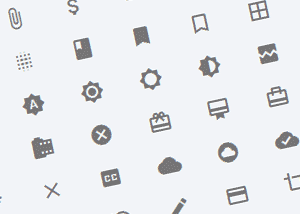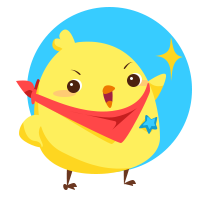### Material design icons2015-12-24

### 《赤壁》标准版/增强版（前导公司·瞬间工作室）

2015-10-20
• 1B （byte 字节） = 8bit
• 1KB（Kilobyte 千字节） = 2^10 B = 1024 B
• 1MB（Megabyte 兆字节） = 2^10 KB = 1024 KB = 2^20 B
• 1GB（Gigabyte 吉字节） = 2^10 MB = 1024 MB = 2^30 B
• 1TB（Trillionbyte 太字节） = 2^10 GB = 1024 GB = 2^40 B
• 1PB（Petabyte 拍字节） = 2^10 TB = 1024 TB = 2^50 B
• 1EB（Exabyte 艾字节） = 2^10 PB = 1024 PB = 2^60 B
• 1ZB（Zettabyte 泽字节） = 2^10 EB = 1024 EB = 2^70 B
• 1YB（YottaByte 尧字节） = 2^10 ZB = 1024 ZB = 2^80 B
• 1BB（Brontobyte / Gegobyte ） = 2^10 YB = 1024 YB = 2^90 B
• 1NB（NonaByte ） = 2^10 BB = 1024 BB = 2^100 B
• 1DB（DoggaByte） = 2^10 NB = 1024 NB = 2^110 B

• 10^0 一
• 10^1 十
• 10^2 百
• 10^3 千
• 10^4 万
• 10^5 十万
• 10^6 百万
• 10^7 千万
• 10^8 亿
• 10^9 十亿（吉）
• 10^10 百亿
• 10^11 千亿
• 10^12 兆（万亿）
• 10^13 十兆
• 10^14 百兆
• 10^15 千兆（拍）
• 10^16 京
• 10^17 十京
• 10^18 百京（艾）
• 10^19 千京
• 10^20 垓
• 10^21 十垓（泽）
• 10^22 百垓
• 10^23 千垓
• 10^24 秭（尧）
• 10^25 十秭
• 10^26 百秭
• 10^27 千秭
• 10^28 穰
• 10^29 十穰
• 10^30 百穰
• 10^31 千穰
• 10^32 沟
• 10^33 十沟
• 10^34 百沟
• 10^35 千沟
• 10^36 涧
• 10^37 十涧
• 10^38 百涧
• 10^39 千涧
• 10^40 正
• 10^41 十正
• 10^42 百正
• 10^43 千正
• 10^44 载
• 10^45 十载
• 10^46 百载
• 10^47 千载
• 10^48 极
• 10^49 十极
• 10^50 百极
• 10^51 千极
• 10^52 恒河沙
• 10^53 十恒河沙
• 10^54 百恒河沙
• 10^55 千恒河沙
• 10^56 阿僧只
• 10^57 十阿僧只
• 10^58 百阿僧只
• 10^59 千阿僧只
• 10^60 那由他
• 10^61 十那由他
• 10^62 百那由他
• 10^63 千那由他
• 10^64 不可思议
• 10^65 十不可思议
• 10^66 百不可思议
• 10^67 千不可思议
• 10^68 无量大数
• 10^69 十无量大数
• 10^70 百无量大数
• 10^71 千无量大数
• 10^72 Espana
• 10^73 十Espana
• 10^74 百Espana
• 10^75 千Espana
• 10^76
• 10^77
• 10^78
• 10^79
• 10^80
• 10^81
• ...... ......
• 10^100 Googol
• ...... ......
• 10^Googol Googolplex
2015-10-18

``````
background: url(http://lao.si/img/logo.png) 50% 50% / cover;
```
```

``````
background: url(http://lao.si/img/logo.png) #000 left/cover fixed;
```
```
2015-10-12

2015-09-30

### 艺术家 马克·J·法拉利 的访谈（Q & A with Mark J. Ferrari）

Mark J. Ferrari 谈谈他的 Color Cycling 作品，具体内容请 >点进来看<

2015-09-28

### 用HTML5实现色彩循环（Old School Color Cycling with HTML5）

2015-09-132015-08-05

2014-11-11

### GraphicsGale 说明书（中文版）

GraphicsGale 是款不错的像素绘画软件。这里是我翻译制作的中文版说明书，希望能够为你提供一些帮助。
2009-12-06